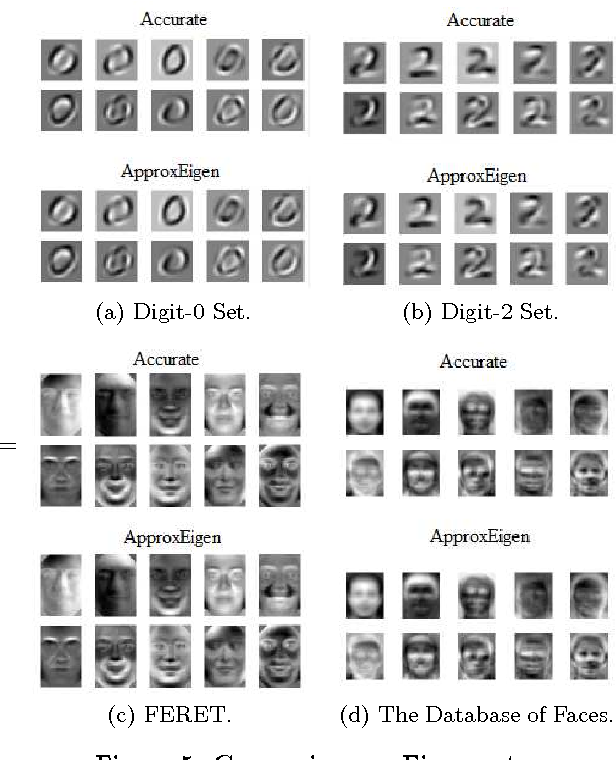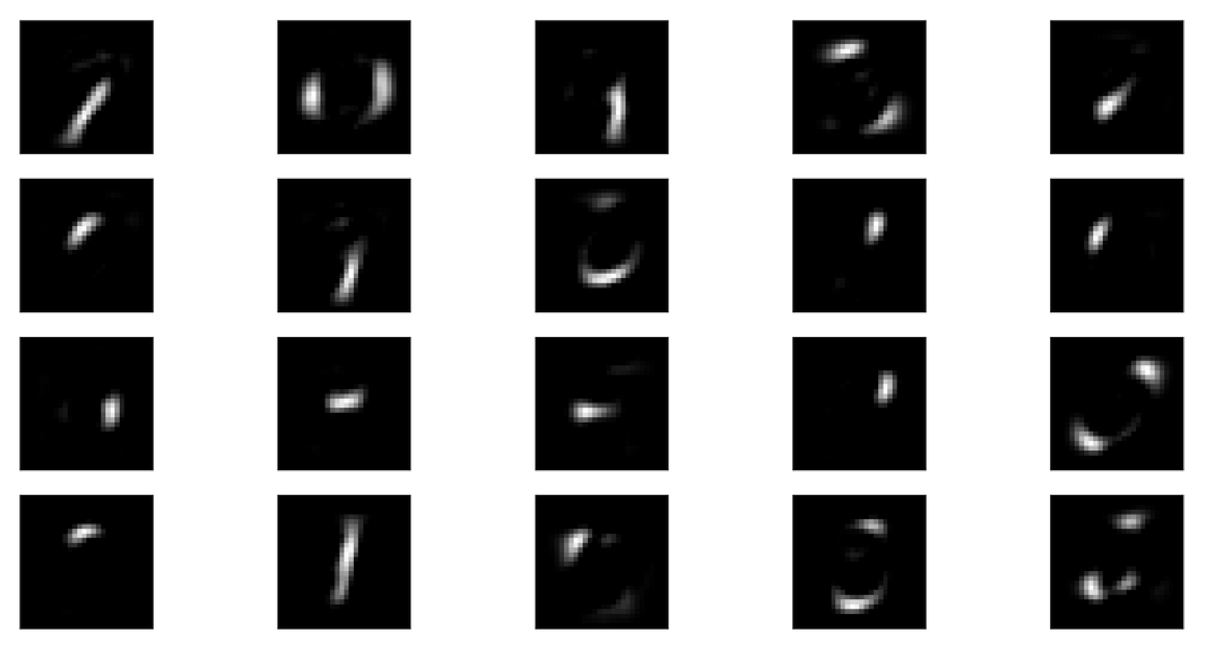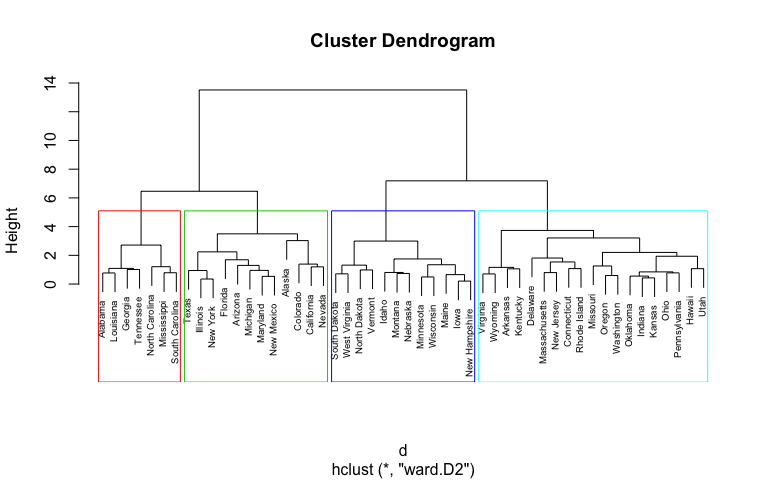6 Matching Annotations
1. Nov 2018
2. 192.168.199.102:5000 192.168.199.102:5000
1. # 三，方法3：MDS

Multi-dimensional scaling (MDS) and Principla Coordinate Analysis(PCoA) are very similar to PCA, except that instead of converting correlations into a 2-D graph, they convert distance among the samples into a 2-D graph.

So, in order to do MDS or PCoA, we have to calculate the distance between Cell1 and Cell2, and distance between Cell1 and Cell3...

• 1 2
• 1 3
• 1 4
• 2 3
• 2 4
• 3 4One very common way to calculate distance between two things is to calculate the Euclidian distance.And once we calculated the distance between every pair of cells, MDS and PCoA would reduce them to a 2-D graph.The bad news is that if we used the Euclidean Distance, the graph would be identical to a PCA graph!!In other words, clustering based on minimizing the linear distances is the same with maximzing the linear correlations.

我想这里也就是为什么，李宏毅老师在 t-SNE 课程一开始时说，其他非监督降维算法都只是专注于【如何让·簇内距小·】，而 t-SNE 还考虑了【如何让·簇间距大·】

也就是说，PCA 的本质（或者叫另一种解释）也只是【找到一种转换函数，他能让原空间中距离近的两点，转换后距离更近】，他压根就没有考虑【簇内or簇外】而是“通杀”所有点。The good news is that there are tons of other ways to measure distance!!!

For example, another way to measure distances between cells is to calcualte between cells is to calculate the average of the absolute value of the log fold changes among genes.Finally， we get a plot different from the PCA plotA biologist might choose to use log fold change to calculate distance because they are frequently interested in log fold changes among genes...

But there are lots of distance to choose from...

1. Manhattan Distance
2. Hamming Distance
3. Great Circle DistanceIn summary:

• PCA creates plots based on correlations among samples;
• MDS and PCoA create plots based on distances among samples#### URL

3. Oct 2018
4. 192.168.199.102:5000 192.168.199.102:5000
1. #### Matrix Factorization

有时候你会有两种 object, 他们之间由某种共通的 latent factor 去操控。

比如我们都会喜欢动漫人物，但我们不是随随便便就喜欢的，而是说在我们自己的性格和动漫人物的性格背后隐含着某个相同的“属性列表”

每个人根据自己的【特点】都可以看成是【属性列表】中的一个向量或是高维空间中的一个点（向量），每个动漫人物也可以根据其【特点】看成是【属性列表】中的一个向量或高维空间中的一个点（向量）。

如果这两个向量很相似的话，那么两者 match，就会产生【喜欢】

假设：

• 已知 $$r_{person}$$ 和 $$r_{idiom}$$ 是这两个向量
• 求：人和动漫人物之间的匹配度（喜欢程度）。

现在：

• 已知 任何动漫人物之间的匹配度（喜欢程度）
• 求：向量$$r_{person}$$ 和 $$r_{idiom}$$

【注意】这个 latent vector 的数目是试出来的，一开始我们并不知道。

比如 latent attribute = ['傲娇', '天然呆']

person A : $$r^A = [0.7, 0.3]$$

character 1: $$r^1 = [0.7, 0.3]$$

$$r^A \cdot r^1 = 0.58$$

• # of Otaku = M
• # of characters = N
• # of latent factor(a vector) = K (means vector r is K dim)

$$X \in R^{M*N} \approx \begin{vmatrix} r^A \\ r^B \\r^C\\.\\.\\. \end{vmatrix}^{M*K} * \begin{vmatrix} r^1 & r^2 & r^3 &.&.&. \end{vmatrix}^{K*N}$$

这个问题可以直接使用 SVD 来解决，虽然SVD分解得到的是三个矩阵，你可以视情况将中间矩阵合并给前面的或后面的

#### MF 处理缺值问题 --- Gradient Descent

上面的是理想情况：table X 中所有的值都完备；

现实情况是：tabel X 通常是缺值的，有很多 Missing value. 那该如何是好呢？

使用 GD，来做，目标函数是：

$$L = \sum_{(i,j)}(r^i \cdot r^j - n_{ij})^2$$

通过对这个目标函数最小化，就可以求出 r.

然后就可以用这些 r 来求出 table 中的每一个值。

MF 的求值函数（table X 的计算函数，我们之前一直假设他是两个 latent vector 的匹配程度）可以考虑更多的因素。他不仅仅可以表示匹配程度

从： $$r^A \cdot r^1 \approx 5$$ 到更精确的： $$r^A \cdot r^1 + b_A + b_1\approx 5$$

• $$b_A$$: 表示他对动漫多感兴趣
• $$b_1$$: 表示这个动漫的推广力度如何

如此新的 GD 优化目标就变成：

$$L = \sum_{(i,j)}(r^i \cdot r^j + b_i + b_j - n_{ij})^2$$

也可以加 L1 - Regularization, 比如 $$r^i, r^j$$ 是 sparse 的---喜好很明确，要么天然呆，要么就是傲娇的，不会有模糊的喜好。

#### MF for Topic analysis

MF 技术用在语义分析就叫做 LSA(latent semantic analysis):

• character -> document
• otakus -> word
• table item -> term frequency of word in this document

注意：通常我们在做 LSA 的时候还会加一步操作，term frequency always weighted by inverse document frequency, 这步操作叫做 TF-IDF.

也就是说，你用作 $$L = \sum_{(i,j)}(r^i \cdot r^j + b_i + b_j - n_{ij})^2$$ 中的 $$n_{ij}$$ 不是原始的某篇文章中的某个单词的出现次数而是出现次数乘以包含这个单词的文章数的倒数亦即，

(n_{ij} = \frac{TF}{IDF})\

如此当我们通过 GD 找到 latent vector 时，这个向量的每一个位表示的是 topics(财经，政治，娱乐，游戏等)

2. ### 从 components-PCA 到 Autoencoder

根据之前通过 SVD 矩阵分解得到的结论： u = w

和公式：$$\hat{x} = \sum_{k=1}^Kc_kw^k \approx x-\bar{x}$$

再结合线性代数的知识，我们可以得到，能让 reconstruction error 最小的 c 就是：

$$c^k = (x-\bar{x})\cdot w^k$$

结合这两个公式，我们就可以找到一个 Autoencoder 结构：

1. $$(x-\bar{x})\cdot w^k = c^k$$
2. $$\sum_{k=1}^Kc^kw^k = \hat{x}$$

$$\begin{vmatrix} x_1 - \bar{x} \\ x_2 - \bar{x} \\ .\\.\\. \end{vmatrix} \Rightarrow \begin{vmatrix} c^1 \\ c^2 \end{vmatrix} \Rightarrow \begin{vmatrix} \bar{x_1} \\ \bar{x_2} \\ .\\.\\. \end{vmatrix}$$

autoencoder 的缺点 --- 无法像 PCA 一样得到完美的数学解

这样一个线性的 autoencoder 可以通过 Gradient Descent 来求解，但是 autoencoder 得到的解只能无限接近 PCA 通过 SVD 或者 拉格朗日乘数法的解，但不可能完全一致，因为 PCA 得到的 W 矩阵是一个列和列之间都相互垂直的矩阵，autoencoder 确实可以得到一个解，但无法保证参数矩阵 W 的列之间相互垂直

autoencoder 的优点 --- 可以 deep，形成非线性函数，面对复杂问题更 power

PCA 只能做压扁不能做拉直

就像下面显示的这样，PCA 处理这类 manifold（卷曲面）的数据是无能为力的。他只能把数据都往某个方向压在一起。而 deep autoencoder 可以处理这类复杂的降维问题：#### how many the principle components?

你要把数据降到几维度，是你可以自己决定的，但是有没有什么比较好的数学依据来hint这件事情呢？

常用的方法是：

$$eigen\ ratio\ = \frac{\lambda_i}{\lambda_1 + \lambda_2 + \lambda_3 + \lambda_4 + \lambda_5 + \lambda_6}$$

• $$\lambda$$ ： eigen value of Cov(x) matrix

我们求解 PCA 的函数的时候给出的结论是：

W 的列是 $$S=Cov(x)$$ 的topmost K 个 eignen values 对应的 eigen vectors.

eigen values 的物理意义是降维后的 z 空间中的数据集在这一维度的 variance。

在决定 z 空间的维度之前，我们引入一个指标： eigen ratio，这个指标有什么用呢？他能帮助我们估算出前多少个 eigen vector 是比较合适的。

假设我们预先希望降维到 6 dimension，那么我们就可以通过之前学到的方法得到 6 个 eigen vector 和 6 个 eigen value, 同时也可以得到 6 个 eigen ratio.

通过这 6 个 eigen ratio 我们就可以看出谁提供的 variance 是非常小的（而我们的目标是找到最大 Variance(z)）。eigen ration 太小表示映射之后的那个维度，所有的点都挤在一起了，他没什么区别度，也就是提供不了太多有用的信息。

之前没有提过，component 的维度应该是与原始 x 样本的维度是一致的，因为你可以把 component 看成是原始维度的一种组合（笔画 <- 像素）。

以宝可梦为例，说明： 如果把原本 6 个维度的宝可梦，降维到4维度，可以发现的是这个 4 个 componets 大概的物理意义是：

1. 1st_component: 强度，对应的原始样本 6 维度都是正系数。
2. 2nd_component: 防御力(牺牲速度)，对应的原始样本中 'Def' 最高，'Speed' 最低（负值）
3. 3rd_component: 特殊防御（牺牲攻击和生命值），对应的原始样本中 'Sp Def' 最高，'HP','Atk'最低（都是负值）
4. 4th_component: 生命值（牺牲攻击力和防御力），对应的原始样本中的'HP'最高， 'Atk' 'Def' 最低（都是负值）

#### 从实际应用看 PCA 得到的 'component'

'component' 不一定是 ‘部分’。

• 对手写数字图片进行降维

• 对人脸图片进行降维上面的图片所展示的似乎异于我们期望看到的啊！

我们一直强调 component 似乎是一种部分与整体的感觉，而这里给出的图片似乎是一种图层的感觉 --- 每一张 'component' 给出的不是笔画和五官，而是完整的数字和脸的不同颜色or阴影。

#### 进一步分析 PCA 得到的 'component' --- 滤镜

提出一种可能性：先看之前的公式，

$$img_9 = c_1w_1 + c_2w_2 + ...$$

由于我们并没有限制，factor 系数的符号（factor 可正可负），所以他极有可能做的一件事情是，

为了生成图片 数字9，我的第一个component可以比较复杂，比如图片数字8，给与其正的factor，第二个component可以是一个0,给与其正的factor，第三个component可以是一个 6，给与其负值的factor

这样 img8 + img0 - img6 看上去就约等于 img9 了。

#### 如何能使得 component 就是‘部分’

PCA 我们使用的分解是 SVD 或者 lagrange multiplier. 这种方法无法保证我得到的 component 和 其对应的 factor 都是正的。于是可以使用 NMF(non-negative matrix factorization)

NMF:

1. forcing all factors be non-negative
2. forcing all components be non-negative

李宏毅老师没有具体讲解这个方法，只列出索引：

Algorithms for non-negative matrix factorization

如下图, NMF 使用的 components 更像是 ‘部分’ 而不是 ‘滤镜’#### URL

5. Sep 2018
6. 192.168.199.102:5000 192.168.199.102:5000
1. #### PCA - Another point of view

Basic Component can ba analogy to sample after projection

可以从比较形象的角度来理解 PCA，之前的角度是从x -> z , PCA 其本质就是一个线性函数，把 x 空间中一点，转换到 z 空间中一点。

$$img_{28*28} \rightarrow x = \begin{vmatrix} 204 \\ 102 \\ 0 \\ 1 \\ . \\ . \\ . \end{vmatrix}^{784*1} \rightarrow z = \begin{vmatrix} 1 \\ 0.5 \\ 0 \\ 1 \\ 0.3 \end{vmatrix}$$

如果把 z 的每一位都看成是某种笔画的系数，那么我们就可以用另外一种方式来表示一张图片：

$$x - \bar{x} \approx c_1u_1 + c_2u_2 + ... + c_ku_k = \hat{x}$$

• $$c_i$$ is the component of x which is an image
• $$u_i$$ is factor of each component
• $$x$$ is img;
• $$\bar{x}$$ is average color degree of pixels;
• $$\hat{x}$$ is the combination of components of a digit.
• $$x-\bar{x}$$ is a normalization to make average degree of color of an image to be 0
• $$||(x-\bar{x})-\hat{x}||_2$$ is reconstruction error

这就提供了另一种看待问题的视角：

• 原始PCA 是通过找到一个方向 w，它能最大化投影之后的Variance
• Components-PCA 是通过找到各个Components的系数，它能最小化 reconstruction error

于是优化目标的函数也就改变了：

• 原始 PCA:

$$argmax_{w_i} Var(x) = \sum_x(x-\bar{x})^2, with\ ||w_i||_2 = 1, and\ w_i \cdot \{w_j\}_{j=1}^{i-1} = 0$$

• Components-PCA:

$$argmin_{u_1, ..., u_K}\sum||(x-\bar{x}) - (\sum_{k=1}^Kc_ku_k)||_2$$

$$\sum_{k=1}^Kc_ku_k = \hat{x}$$

可以证明的是：这个 $$\{u_k\}_{k=1}^K$$ 就是我们要找的 $$\{w_k\}_{k=1}^K$$

$$\begin{vmatrix} z_1 \\ z_2 \\ . \\. \\. \\z_K \end{vmatrix} = \begin{vmatrix} w_1 \\ w_2 \\ . \\. \\. \\w_K \end{vmatrix} x = \begin{vmatrix} u_1 \\ u_2 \\ . \\. \\. \\u_K \end{vmatrix} x$$

#### 证明 u = w

$$x^1 - \bar{x} \approx c_1^1u^1 + c_2^1u^2 + ...$$

$$x^2 - \bar{x} \approx c_1^2u^1 + c_2^2u^2 + ...$$

$$x^3 - \bar{x} \approx c_1^3u^1 + c_2^3u^2 + ...$$

• $$x^i$$ : 第 i 个样本
• $$c_k^i$$ : 第 i 个样本的第 k 个 component 的系数（权重）
• $$u^k$$: 第 k 个 component

【注意】component 对任意样本都是一样的，样本之间不同的是component的系数（权重）

我们可以把上面 3 个式子组合成矩阵的形式：

##### 对于单个样本

可以写成 向量 = 矩阵 * 向量

$$c_1^2u^1 + c_2^2u^2 + ... = \sum_{k=1}^Kc_k^1u^k = [u^1, u^2, ...] \cdot \begin{vmatrix}c_1^1\\c_2^1\\.\\.\\.]\end{vmatrix}$$

##### 把所有样本都组合起来

可以写成 矩阵 = 矩阵 * 矩阵

$$\underbrace{[x^1 - \bar{x}, x^2-\bar{x}, x^3-\bar{x}, ...]}_{\text{dataset:X}} \approx \underbrace{[u^1, u^2, ...]}_{\text{components matrix}} \cdot \underbrace{ \begin{vmatrix} c_1^1 & c_1^2 & c_1^3\\ c_1^1 & c_1^2 & c_1^3\\ c_1^1 & c_1^2 & c_1^3\\ .&.&.\\ .&.&.\\ .&.&. \end{vmatrix}}_{\text{factor matrix}} \text{how to find the best u to make two side of approx as near as possible?}$$

我们可以使用 SVD 矩阵分解，对 X 进行分解：

X 可以分解成3个矩阵的乘积：

$$X_{m*n} \approx U_{m*k} \Sigma_{k*k}V_{k*n}$$

• m : the dimension of one sample
• n : the size of dataset
• k : number of components

这里直接给出答案，具体过程见线性代数课程。

K columns of U: a set of orthonormal eigen vectors corresponding to the k largest eigenvalues of $$XX^T$$

这个 $$XX^T$$ 就是 $$Cov(x)$$ , 因为这里的 X 是由 $$x - \bar{x}$$ 得到的。所以，u = w。

再回忆下我们对于 u w 和 z 的定义：

• u : components of x (eg, 数字的笔画)
• w : 线性函数矩阵 W 用于做 $$z = W^Tx$$ 投影
• z : 投影之后的“新”的样本

启示：PCA 找出的那些 w(转换矩阵 W 的 columns) 就是 components

2. #### Dimension Reduction 为什么可能是有用的？

一，例子1 为什么 Dimension Reduction 可能是有用的，如下图示：如果我们用 3 dimension 的样本维度去描述他其实是非常浪费的，因为很明显他是卷曲在一起的，如果我们有办法把他展平，不同类型（不同颜色代表不同类型）就很容易区隔开，而展平之后我们只需要 2 dimension 的样本维度。所以根本不需要把这个问题放在 3d 中去考虑，2d 就可以了。二，例子2

在手写数字辨识的任务中，你或许根本用不到 28*28 的维度，比如数字 3 他可能有很多形态，但其实大部分都是旋转一下角度而已，所以对于辨识 3 这件事可能需要的维度仅仅是一个旋转角度而已（1维度）

#### 如何做 dimension reduction 呢？

对数据降维就是找一个function

$$f(x) \rightarrow x', dim(x) >> dim(x')$$

#### 二，方法2：PCA

##### 基本原理

PCA 的本质就是通过一个一次线性函数 $$W^Tx$$把高维度的数据样本转换为低维度的数据样本。就像 $$w^Tx$$ 如果$$W^T$$矩阵只有一行，这个函数就是两个向量的内积 --- 结果是一个（一维）数值，如果 $$W^T$$ 是一个矩阵，那么 $$W^T$$ 有多少行最终内积结果就有多少维

$$R_W^{1*N} \cdot R_x^{N*1} = R_{x'}^{1*1} R_W^{2*N} \cdot R_x^{N*1} = R_{x'}^{2*1} ...$$

$$f(x) \rightarrow x', dim(x) >> dim(x')$$

$$z = Wx, to\ find\ 'W'$$

##### 找到优化问题 --- projection has max variance

该如何找到 W 呢，从最简单的开始，我们假设 :

• reduce x to 1 D vector z, means that 'z' is a digit
• 'W' matrix only have ONE row
• this row's L2 norm is equal to 1: $$||w^1||_2=1$$.

如此一来，'z' 就可以看做是 'x' 在 'w' 方向上的投影（内积的几何意义）

所有的 x: $$x^1, x^2, x^3,...$$ 都在 w 方向上做投影就得到了 z: $$z^1, z^2, z^3,...$$ , 而我们要做的就是从：

如何找到 W 变成 找到一个 x 的更好的投影方向

we want the variance of $$z_1$$ as large as possible.

variance 在图像上的表现就是，投影之后的 z 的取值范围（值域）取值范围越大variance越大

对于投影到一维空间，找到最佳的 w('w' is a row vector) 使得 x('x' is N dimension) 投影到该方向得到的 z ('z' is a digit)的 variance 是最大的， 用公式表示就是：

$$find\ a\ w\ to\ maximize:$$

$$Var(z_1) = \sum_{z_1}(z_1 - \bar{z_1})^2)$$

$$with\ constaint\ :\ ||w_1||_2=1$$

对于投影到二维（甚至多维）空间，我们要给每个后面的w一个限制就是: 后面的 w(n+1 th row) 要跟前面的所有 w({1~n} rows) 相互垂直, $$w_{n+1} \cdot \{w_{i}\}_{i=1}^n = 0$$.

整体来看就是：

the 1st row of W should be:

$$w_1 = argmax(Var(z_1) = \sum_{z_1}(z_1 - \bar{z_1})^2)$$

$$constraint:\ ||w_1||_2=1$$

the 2nd row of W should be:

$$w_2 = argmax(Var(z_2) = \sum_{z_2}(z_2 - \bar{z_2})^2)$$

$$constraint:\ ||w_2||_2=1, w_2 \cdot w_1 = 0$$

the 3rd row of W should be:

$$w_3 = argmax(Var(z_3) = \sum_{z_3}(z_3 - \bar{z_3})^2)$$

$$constraint:\ ||w_3||_2=1, w_3 \cdot w_1 = 0, w_3 \cdot w_2 = 0$$

.......

then the W should be:

$$W = \begin{vmatrix} (w_1)T \\ (w_2)T \\ . \\ . \\ . \end{vmatrix}\quad$$

这里 W 会是一个 Orthogonal matrix, 因为每一行的 norm 都等于1，并且行行之间相互垂直。

##### 求解这个带限制条件优化问题 ---- 拉格朗日乘数法

Lagrange multiplier 可以把带限制条件的优化问题转换>为无限制优化问题： target - a(zero_form of >constraint1) - b(zero_form of constraint2)

无限制的优化问题，就可以通过偏微分=0来找到最优解

如果 W 矩阵只有一行

$$one\ x\ one\ z_1 \bar{z_1} = \sum{z_1} = \sum{w_1 \cdot x} = w_1 \cdot \sum x = w_1 \cdot \bar{x} Var(z_1) = \sum_{z_1}(z_1 - \bar{z_1})^2 = \sum_x(w_1 \cdot x - w_1 \cdot \bar{x})^2 = \sum_x(w_1 \cdot (x-\bar{x}))^2$$

对于 $$w \cdot (x - \bar{x})$$, 他是两个向量的内积，对于这种内积的平方，要注意如下的惯用转换公式（值得记住）。

$$(a \cdot b)^2 = (a^Tb)^2$$

因为 $$a \cdot b$$ 是内积，是一个数值，数值的平方无关乎顺序，所以可以写成：

$$(a^Tb)^2 = a^Tba^Tb$$

因为 $$a \cdot b$$ 是内积，是一个数值，数值的转置还是自身，所以可以写成：

$$(a^Tba^Tb = a^Tb(a^Tb)^T$$

化简之后：

$$a^Tb(a^Tb)^T = a^Tbb^Ta$$

所以之前 var 的式子可以化简为：

$$Var(z_1) = \sum_{z_1}(z_1 - \bar{z_1})^2 = \sum_x(w_1 \cdot x - w_1 \cdot \bar{x})^2 = \sum_x(w_1 \cdot (x-\bar{x}))^2 = \sum_x(w_1)^T(x-\bar{x})(x-\bar{x})^Tw_1$$

因为是对 x 来求和，所以 w 可以提出去：

$$Var(z_1) = \sum_{z_1}(z_1 - \bar{z_1})^2 = \sum_x(w_1 \cdot x - w_1 \cdot \bar{x})^2 = \sum_x(w_1 \cdot (x-\bar{x}))^2 = \sum_x(w_1)^T(x-\bar{x})(x-\bar{x})^Tw_1 = (w_1)^T(\sum_x(x-\bar{x})(x-\bar{x})^T)w_1$$

其中 $$\sum_x(x-\bar{x})(x-\bar{x})^T$$ 是什么，这个是 x 向量（样本都是向量）的 covariance matrix. covariance 是对称且半正定对的， 也就是说所有的 eigenvalues 都是非负的

$$Var(z_1) = \sum_{z_1}(z_1 - \bar{z_1})^2 = \sum_x(w_1 \cdot x - w_1 \cdot \bar{x})^2 = \sum_x(w_1 \cdot (x-\bar{x}))^2 = \sum_x(w_1)^T(x-\bar{x})(x-\bar{x})^Tw_1 = (w_1)^T(\sum_x(x-\bar{x})(x-\bar{x})^T)w_1 = (w_1)^TCov(x)w_1$$

我们设 $$S = Cov(x)$$ ,于是我们得到了一个新的优化问题：

find w1 maximizing:

$$w_1^T S w_1, with\ constraint\ ||w_1||_2 = 1, means\ w_1^Tw_1 = 1$$

Lagrange multiplier is target - zero_form of constraints

之前说明过 $$w_1$$ 是 W 矩阵的第一个row，再次提醒下。

$$g(w_1) =w_1^TSw_1 - \alpha(w_1^Tw_1 - 1)$$

$$\frac{\partial g(w_1)}{\partial w_{11}} = 0$$

$$\frac{\partial g(w_1)}{\partial w_{12}} = 0$$

....

通过对 Lagrange 式子 $$g(w_1)$$ 的微分为0来求最大最优的w，可以得到如下化简后的式子：

$$Sw_1 - \alpha w_1 = 0$$

$$Sw_1 = \alpha w_1$$

这种形式我们就熟悉了，$$w_1$$ 是 S 的 eigen vector

但是这样的 w1 有很多，因为 S 的 eigen vector 有很多，所以 w1 的潜在选择也很多，哪一个是最好的选择呢？通过我们的优化目标来判断。

$$w_1^TSw_1 = \alpha w_1^Tw_1 = \alpha$$

而这里的 $$\alpha$$ 是 eigen value,

所以，因为我们要最大化的目标最后等于 alpha, 而 alpha 是 eigen value, 所以我们选择最大的 eigen value, 一个 eigen value 对应一个 eigen vector, 所以最优的 w1 就是最大的 eigen value 对应的那个 eigen vector.

之前分析过， Find w2 的情况与 Find w1 略有不同，不同在优化问题的限制条件多出一个与之前所有的w都垂直，那么就是：

$$Find\ w_2\ maximizing: w_2^TSw_2, w_2Tw_2=1, w_2^Tw_1=0$$

按照 目标函数 - 系数*zero_form of 限制条件 的拉格朗日使用方法，可以得到如下无限制条件的优化问题：

如果 W 矩阵有多行

对于 W 矩阵有多行的情况，优化目标函数需要改变， 要增加限制条件，如果不加改变的话你得到的 wn 永远与 w1 一样，增加的这个限制条件是：后面的每个w都要与前面的所有w相互垂直 --- 内积为0

$$Find\ w_2\ maximizing:$$

$$g(w_2) = w_2^TSw_2 - \alpha(w_2^Tw_2-1)-\beta(w_2^Tw_1-0)$$

同样的通过微分等于0来求无限制条件的优化问题：

$$\frac{\partial g(w_1)}{\partial w_{11}} = 0$$

$$\frac{\partial g(w_1)}{\partial w_{12}} = 0$$

化简上述结果可以得到：

$$Sw_2 - \alpha w_2 - \beta w_1 = 0$$

上述等式两边同时乘以 w1：

$$w_1^TSw_2 - \alpha w_1^Tw_2 - \beta w_1^Tw_1 = 0$$

$$w_1^TSw_2 - \alpha * 0 - \beta * 1 = 0$$

因为 $$w_1^TSw_2$$ 本身是一个 向量矩阵向量 的形式，他是一个数值，数值的转置还是自身：

$$w_1^TSw_2 = (w_1STw_2)^T = w_2^TS^Tw_1$$

因为 S 是 Covariance Matrix of x, 是对称的，所以 $$S^T=S$$

$$w_2^TS^Tw_1= w_2^TSw_1$$

使用 w1 的结论，$$Sw_1 = \lambda_1w_1$$, 所以有

$$w_2^TSw_1=\lambda_1w_2^Tw_1=0$$

所以，综合起来看：

$$w_1^TSw_2 - \alpha w_1^Tw_2 - \beta w_1^Tw_1 = 0$$

$$w_1^TSw_2 - \alpha * 0 - \beta * 1 = 0$$

$$0 - \alpha * 0 - \beta * 1 = 0$$

所以：

$$\beta=0$$

所以：

$$Sw_2 - \alpha w_2= 0$$

$$Sw_2 = \alpha w_2$$

由于 w2 也是 eigen vector, 同样有很多选择，与 w1 选择的依据一样，我们只能选择剩下最大的那个 eigenvalue of S 对应的 eigenvector

so，结论是：

$$z = Wx$$

$$W = \begin{vmatrix} (w_1)T \\ (w_2)T \\ . \\ . \\ . \end{vmatrix}\quad$$

$$W = \begin{vmatrix} 1st\ largest\ eigenvalues'\ eigenvector\ of\ Cov(x) \\ 2nd\ largest\ eigenvalues'\ eigenvector\ of\ Cov(x) \\ 3rd\ largest\ eigenvalues'\ eigenvector\ of\ Cov(x) \\ ...\end{vmatrix}$$

#### PCA 的副产品 --- 降维后数据点的各个维度互相垂直

数据点的各个维度互相垂直

用数学语言来表述就是

数据点的协方差矩阵是一个 Diagonal matrix

也就是

数据点的各个维度之间没有 correlation

这样有什么作用呢？

一个很好的作用是，原来的 data 经过 PCA 之后输出的新的 data 可以接其他较为简单的（或是对数据点维度要求无 correlation 的）model ---> eg. Naive Bayes.

##### 如何证明呢？

$$Cov(z) = \sum(z - \bar{z})(z-\bar{z})^T$$

这一项，可以可以展开合并后成为下面这种形式：

$$Cov(z) = \sum(z - \bar{z})(z-\bar{z})^T = WSW^T,\ \ S = Cov(x)$$

$$W^T=\begin{vmatrix} w_1 \\ .\\.\\.\\ w_k \end{vmatrix}^T= [w_1, ..., w_k]$$

注意转置之前 w1 是 W矩阵的行向量，转置后，w1 是 W^T 的 列向量：

$$\begin{vmatrix} 1 & 2 & 3 & \rightarrow w_1\\ 4 & 5 & 6 & \rightarrow w_2 \\ 7 & 8 & 9 & \rightarrow w_3 \end{vmatrix}^T \rightarrow \begin{vmatrix} 1 & 4 & 7 \\ 2 & 5 & 8 \\ 3 & 6 & 9 \\ \downarrow & \downarrow & \downarrow \\ w_1 & w_2 & w_3 \end{vmatrix}$$

$$WSW^T = WS[w_1, ..., w_k] = W[Sw_1, ..., Sw_k]$$

利用之前的结论：

$$Sw_1 = \lambda_1 w_1$$

$$W[Sw_1, ..., Sw_k] = W[\lambda_1 w_1, ..., \lambda_k w_k] = [\lambda_1Ww_1, ..., \lmabda_kWw_k] = [\lambda_1e_1, ..., \lambda_ke_k]$$

其中 $$e_i$$ 是指第 $$i$$ 位为 1， 其余位都为 0 的列向量。

所以 $$Cov(z)$$ 就是一个 Diagonal matrix.

#### 总结

PCA 关键词：

• linear function, projection
• maximize Variance of z
• Covariance Matrix of x
• eigenvector of Covariance Matrix

一个重要的结论需要记住： Covariance Matrix is a symmetric, positive-semidefinite matrix, so it has non-negative eigenvalues

一个重要的技术：

带限制条件优化

--- Lagrange multiplier ---> 无限制条件优化

--- partial dirivitive = 0 --->

求解。

一个重要的机器学习常识：

数据点的各个维度互相垂直

用数学语言来表述就是

数据点的协方差矩阵是一个 Diagonal matrix

也就是

数据点的各个维度之间没有 correlation

3. ### Unsupervised learning - Linear Methods

#### Ordinary Clustering

1. random choose K data point as initial centers
2. compute the distance of each sample, choose the label of center which has smallest distance between
3. update the cluster who add-in new element by

$$b_i^n = 1\ if\ x^n\ is\ most\ close\ to\ c_i,\ else\ 0$$

$$c_i = \sum_{x^n}b_i^nx^n/\sum_{x^n}b_i^n$$

#### Hierarchical Agglomerative Clustering(HAC)

一， build a tree

1. 所有 example 两两计算相似度(similarity)
2. 选相似度最高的两个，并把它们 merge 在一起（具体方式可以使用平均 $$v_{new} = \frac{1}{2}(v_1 + v_2)$$）,这样就得到一个同维度的新向量样本
3. 然后再重复步骤2，choose -> merge -> new vector

把原来的样本都看作叶子节点，merge 后的样本看成父亲节点，这样一直做下去直到产生root，这样就构成了一棵树。

二，pick a threshold

这一刀切在哪里就决定了你有多少个分类。比如下图所示，在第二层节点下切一刀就产生出四个簇。#### Distribution Representation

如果只做 clustering 就像是我们做的非黑即白假设一样，未免过于武断，所以这里引入了Distribution representation .

英雄包含7个种类： [强化系，放出系，变化系，操作系，具现化系，特质系]

• Cluster Representation: clustering 方法要求所有样本都属于并且必须只能属于一个簇，

$$Noxus\ of\ LOL \in Strength$$

但有时候这样做未免武断，所以考虑用概率分布的形式来表示一个样本，同时这种表示样本的方法也可以用在 Dimension reduction 中。

• Distribution Representation: [0.7, 0.25, 0.05, 0.0, 0.0, 0.0, 0.0]

Distribution Representation 还一个作用，就是用作降维。这个东西与 Dimension reduction 有莫大的关系，对于一个有很高维度的向量样本而言，如果我们用一些特点来描述他，那就可以大幅度的减少样本的维度。